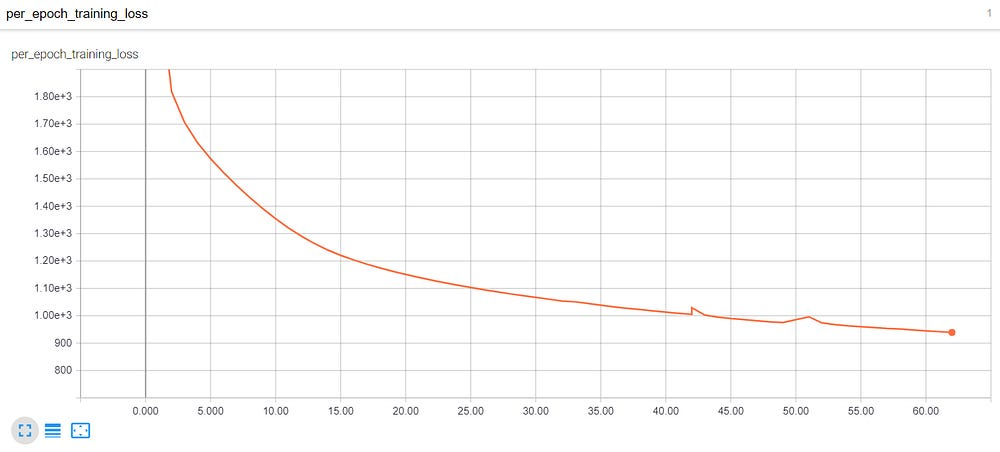# Tensorflow eager for language generation

Text generation is a task in which we generate sentences based on the probability distribution of words learnt by the model from the training data. We trained our model on Leo Tolstoy’s War and Peace so it can understand the style of his writing and try to generate new sentences.

War and Peace is a difficult text to model upon as out of the 38,000 odd total vocabulary size the number of words that repeated only once was around 18,000.

Tensorflow Eager provides the flexibility to use Tensorflow without using graphs, so we can build graphs on the fly during run time. Tensorflow eager provides multiple advantages and below are the few as listed on Tf website

• An intuitive interface — Structure your code naturally and use Python data structures. Quickly iterate on small models and small data.
• Easier debugging — Call ops directly to inspect running models and test changes. Use standard Python debugging tools for immediate error reporting.
• Natural control flow — Use Python control flow instead of graph control flow, simplifying the specification of dynamic models.

And i have never used PyTorch so cannot comment on the performance comparison between Tensorflow Eager and PyTorch.

Now lets dive into the code

`#loading librariesimport numpy as npimport mathimport randomimport warningswarnings.filterwarnings("ignore")import tensorflow as tftf.enable_eager_execution()batch_size = 32word_embedding_size = 80from gensim.models import Word2Vec`

Text Pre-processing and train test split

`f = open('war_peace.txt', 'r',encoding='utf8')x = f.read()f.close()x=x.split("\n\n")for i in range(0,len(x)):    x[i]=x[i].replace("\n"," ")new_text=[]for i in range(0,len(x)):    text=x[i].split(". ")for j in range(0,len(text)):        new_text.append(text[j])for i in range(0,len(new_text)):    new_text[i]=new_text[i].lower()new_txt = []for i in range(0,len(new_text)):if(78>len(new_text[i].split(" "))):        new_txt.append(new_text[i])  train_data = []for i in range(0,len(new_txt)):if len(new_text[i].split())>1:        train_data.append('<start> '+ new_txt[i] + " <end>")for i in range(0,len(train_data)):    train_data[i]=train_data[i].split(" ")`
`test_data = train_data[26773:]train_data = train_data[:26773]`

built the word2vec model once and then saved it so as to maintain training consistency after PC reboot

`model_w2v = Word2Vec.load("word2vec.model")model_w2v_tes = Word2Vec.load("word2vec_tes.model")vocab = list(model_w2v.wv.vocab)a = list(range(1,len(vocab)+1))vocab_test = list(model_w2v_tes.wv.vocab)vocab_dict = dict(zip(a,vocab))vocab_dict_inv = dict(zip(vocab,a))dif = list(set(vocab_test) - set(list(model_w2v.wv.vocab)))`

function to convert the data into fixed length

`def conv(data):    train = np.zeros([len(data),80,1],dtype=np.int64)    for i in range(len(data)):        for j in range(len(data[i])):            try:                train[i][j] = vocab_dict_inv[data[i][j]]            except KeyError:                train[i][j] = vocab_dict_inv[random.choice(dif)]    return train`
`## invoking the conv function`
`final_train = conv(train_data)final_test = conv(test_data)`

instead of padding all the words to max length in the dataset just padding the sentences to max length in batch.
this function also converts words to word2vec embeddings

`def proces_on_batch(data):    data_update = []    data = np.array(data)    for i in range(len(data)):        data_update.append(list(np.array(data[i]).reshape())[:list(np.array(data[i]).reshape()).index(4) + 1])    max_len_in_batch = len(max(data_update, key=len))    train = np.zeros([batch_size,max_len_in_batch,word_embedding_size])    target = np.zeros([batch_size,max_len_in_batch,len(vocab)+1])    for k in range(0,batch_size):        for m in range(max_len_in_batch):            target[k][m] = 1    zeros = np.zeros([word_embedding_size])    for i in range(len(data_update)):        for j in range(len(data_update[i])-1):            target[i][j] = 0            target[i][j][data_update[i][j+1]] = 1            train[i][j] = model_w2v.wv[vocab_dict[data_update[i][j]]]    return train,target`

creating the data input pipe line and creating the iterators

`dataset = tf.data.Dataset.from_tensor_slices(final_train)test_dataset = tf.data.Dataset.from_tensor_slices(final_test)dataset = dataset.batch(batch_size)test_dataset = test_dataset.batch(batch_size)test_iterator = test_dataset.make_one_shot_iterator()iterator = dataset.make_one_shot_iterator()`

initializing the optimizer & LSTM states for use during inference

`lstm_1_ht = tf.contrib.eager.Variable(np.zeros([1,128]),dtype=tf.float32)lstm_1_ct = tf.contrib.eager.Variable(np.zeros([1,128]),dtype=tf.float32)lstm_2_ht = tf.contrib.eager.Variable(np.zeros([1,128]),dtype=tf.float32)lstm_2_ct = tf.contrib.eager.Variable(np.zeros([1,128]),dtype=tf.float32)`

keras.Model class definition and all the variables in this class will be trained by the optimizer

`class language_model(tf.keras.Model):    def __init__(self):        super(tf.keras.Model,self).__init__()        self.LSTM_1 = tf.keras.layers.LSTM(128,return_sequences = True,                                        recurrent_initializer= tf.keras.initializers.truncated_normal(stddev=0.1),                                           recurrent_regularizer = tf.keras.regularizers.l2(0.01),                                           kernel_initializer=tf.keras.initializers.truncated_normal(stddev=0.1),                                          bias_initializer='zeros',kernel_regularizer=tf.keras.regularizers.l2(0.01),                                           bias_regularizer = tf.keras.regularizers.l2(0.01),return_state = True)        self.LSTM_2 = tf.keras.layers.LSTM(128,return_sequences = True,                                           recurrent_initializer = tf.keras.initializers.truncated_normal(stddev=0.1),                                           recurrent_regularizer = tf.keras.regularizers.l2(0.01),                                           kernel_initializer=tf.keras.initializers.truncated_normal(stddev=0.1),                                          bias_initializer='zeros',kernel_regularizer=tf.keras.regularizers.l2(0.01),                                           bias_regularizer = tf.keras.regularizers.l2(0.01),return_state = True)        self.out = tf.keras.layers.TimeDistributed(tf.keras.layers.Dense(len(vocab)+1,                                                kernel_initializer=tf.keras.initializers.truncated_normal(stddev=0.1),                                                bias_initializer='zeros',                                                kernel_regularizer=tf.keras.regularizers.l2(0.01),                                               bias_regularizer = tf.keras.regularizers.l2(0.01)))        self.lstm1_ht = tf.contrib.eager.Variable(np.zeros([batch_size,128]),dtype=tf.float32,name='LSTM_1_ht')        self.lstm1_ct = tf.contrib.eager.Variable(np.zeros([batch_size,128]),dtype=tf.float32,name='LSTM_1_ct')        self.lstm2_ht = tf.contrib.eager.Variable(np.zeros([batch_size,128]),dtype=tf.float32,name='LSTM_2_ht')        self.lstm2_ct = tf.contrib.eager.Variable(np.zeros([batch_size,128]),dtype=tf.float32,name='LSTM_2_ct')    def main_model(self,train_values,state):        global lstm_1_ht        global lstm_1_ct        global lstm_2_ht        global lstm_2_ct        if state == "train":            x,_,_ = self.LSTM_1(train_values,initial_state = [self.lstm1_ht,self.lstm1_ct] )            x,_,_ = self.LSTM_2(x,initial_state = [self.lstm2_ht,self.lstm2_ct] )            x = self.out(x)            return x        else:            x,lstm_1_ht,lstm_1_ct = self.LSTM_1(train_values,initial_state = [lstm_1_ht,lstm_1_ct])            x,lstm_2_ht,lstm_2_ct = self.LSTM_2(x,initial_state = [lstm_2_ht,lstm_2_ct] )            x = self.out(x)            return xmodel = language_model()`

loss function definition need to use gradient tape which calculating the gradients and then passing the gradients to optimizer

`def loss_fun(train_batch,target):    with tf.GradientTape() as t:        loss = tf.reduce_mean(tf.nn.softmax_cross_entropy_with_logits_v2(labels=target,logits=model.main_model(train_batch,"train")))    grads = t.gradient(loss,model.variables)    optimzer.apply_gradients(zip(grads,model.variables))    return loss`

restoring the weights if required

`model.main_model(tf.zeros([batch_size,60,word_embedding_size]),state="train") ## just for the LSTM weights to be initialized so that values can be restoredtf.contrib.eager.Saver.restore(file_prefix='jai_model_v2/weights61',self=tf.contrib.eager.Saver(var_list=list(model.variables)))global_step = tf.train.get_or_create_global_step()global_step.assign(50)`

Creating a summary writer for writing files for tensorboard

`writer = tf.contrib.summary.create_file_writer('loss_lm')writer.set_as_default()`
`## function to capture loss (scalar summary) for tensorboard visualizationdef loss_viz(epoch_training_loss):    with tf.contrib.summary.always_record_summaries():        tf.contrib.summary.scalar("per_epoch_training_loss",epoch_training_loss)`

below is the training loop.
once the iterator is done with one batch it will throw a OutOfRange exception will catch the exception and restart iterator.
initializable iterator is not supported in tensorflow eager.

`iterator = dataset.make_one_shot_iterator()total_loss = 0i = 50while i < 62:    try:        train_batch = iterator.get_next()        train_batch,target = proces_on_batch(train_batch)`
`train_batch = tf.cast(train_batch,dtype=tf.float32)        target = tf.cast(target,dtype=tf.float32)`
`loss = loss_fun(train_batch,target)        total_loss += np.array(loss)    except tf.errors.OutOfRangeError:        print("loss for epoch ",i," is: ",total_loss)        iterator = dataset.make_one_shot_iterator()        global_step.assign_add(1)        loss_viz(total_loss)        tf.contrib.eager.Saver.save(file_prefix='jai_model_v2/weights' + str(i),self=tf.contrib.eager.Saver(var_list=list(model.variables)))        i = i + 1        total_loss = 0`

below is the inference loop

`#### initializing the cell state and hidden state ####lstm_1_ht = tf.reshape(model.lstm1_ht,shape=[1,128])lstm_1_ct = tf.reshape(model.lstm1_ct,shape=[1,128])lstm_2_ht = tf.reshape(model.lstm2_ht,shape=[1,128])lstm_2_ct = tf.reshape(model.lstm2_ct,shape=[1,128])h = 1`
`#### initializing with <start> tokencurrent_word = (model_w2v.wv['<start>']).reshape([1,1,word_embedding_size])`
`#### inference functiondef inference(current_word,search):    global h    current_word = model.main_model(current_word,"inference")    #### condition for greedy or random search    if search == 'greedy':        current_word = np.random.choice(np.argsort(np.array(((tf.nn.softmax(current_word)))))[-1:])    else:        current_word = np.random.choice(np.argsort(np.array(((tf.nn.softmax(current_word)))))[-5:])    if current_word == 0:        current_word = np.zeros([1,1,word_embedding_size])        print("<pad>",end=" ")    else:        current_word = vocab_dict[current_word]        if current_word == "<end>":            print("\n")            lstm_1_ht = tf.reshape(model.lstm1_ht[h],shape=[1,128])            lstm_1_ct = tf.reshape(model.lstm1_ct[h],shape=[1,128])            lstm_2_ht = tf.reshape(model.lstm2_ht[h],shape=[1,128])            lstm_2_ct = tf.reshape(model.lstm2_ct[h],shape=[1,128])            h += 1`
`else:            print(current_word,end=" ")        current_word = (model_w2v.wv[current_word]).reshape([1,1,word_embedding_size])    return current_word`

random search inference & Greedy Search

`for i in range(0,100):    current_word = inference(tf.convert_to_tensor(current_word,dtype=tf.float32),search = 'random')`
`for i in range(0,100):    current_word = inference(tf.convert_to_tensor(current_word,dtype=tf.float32),search = "greedy")`

Loss vs epoch plot as captured on tensorboard

Generating Text using Random search and Greedy Search (if any one has efficient beam search implementation please share)